# 4. Find the first three nonzero terms in a power series expansion about xo = 0...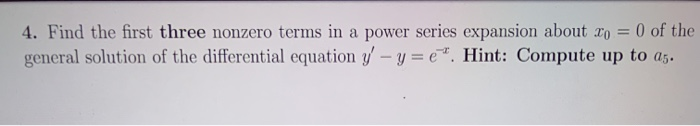4. Find the first three nonzero terms in a power series expansion about xo = 0 of the general solution of the differential equation y' - y=e". Hint: Compute up to 25.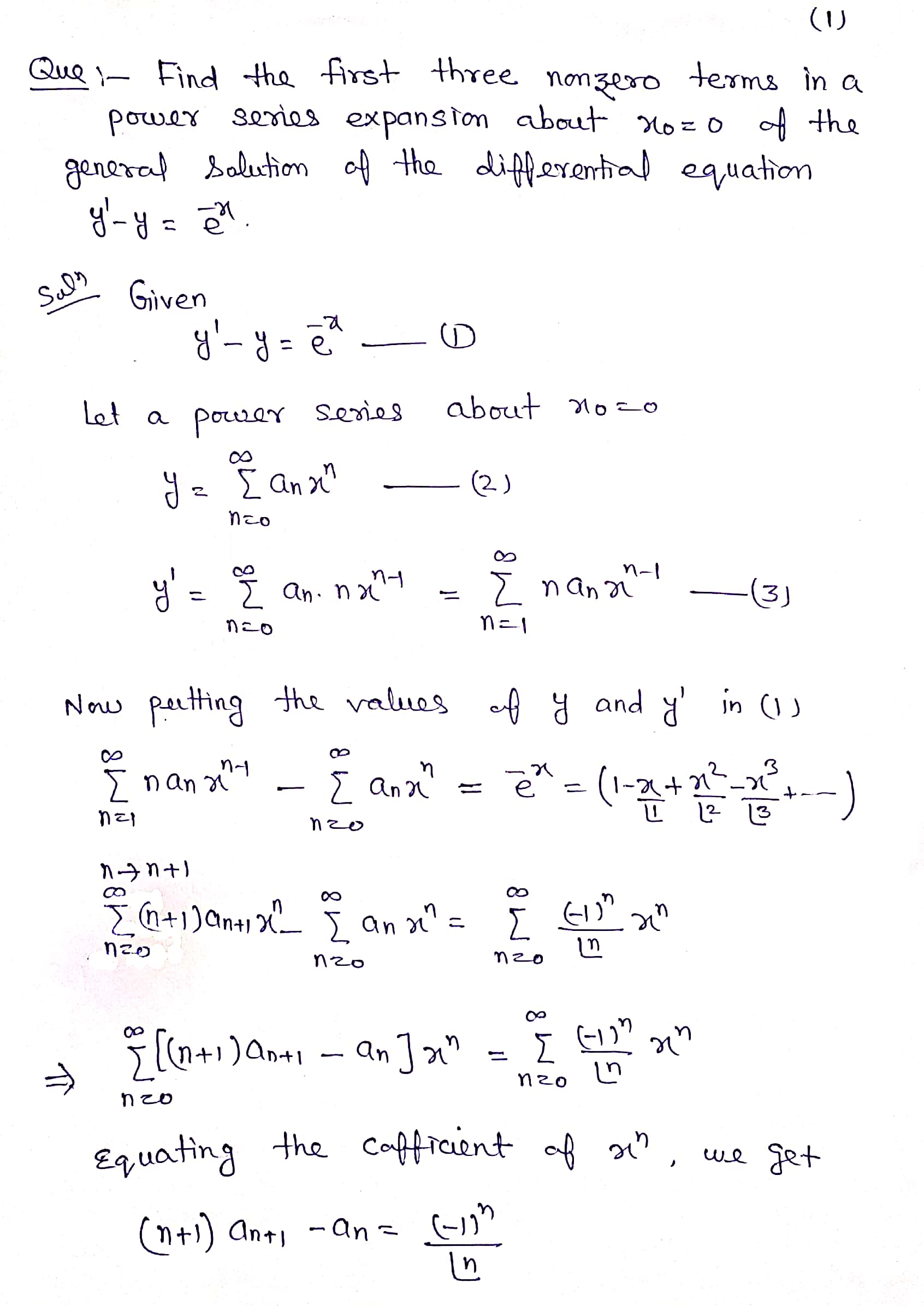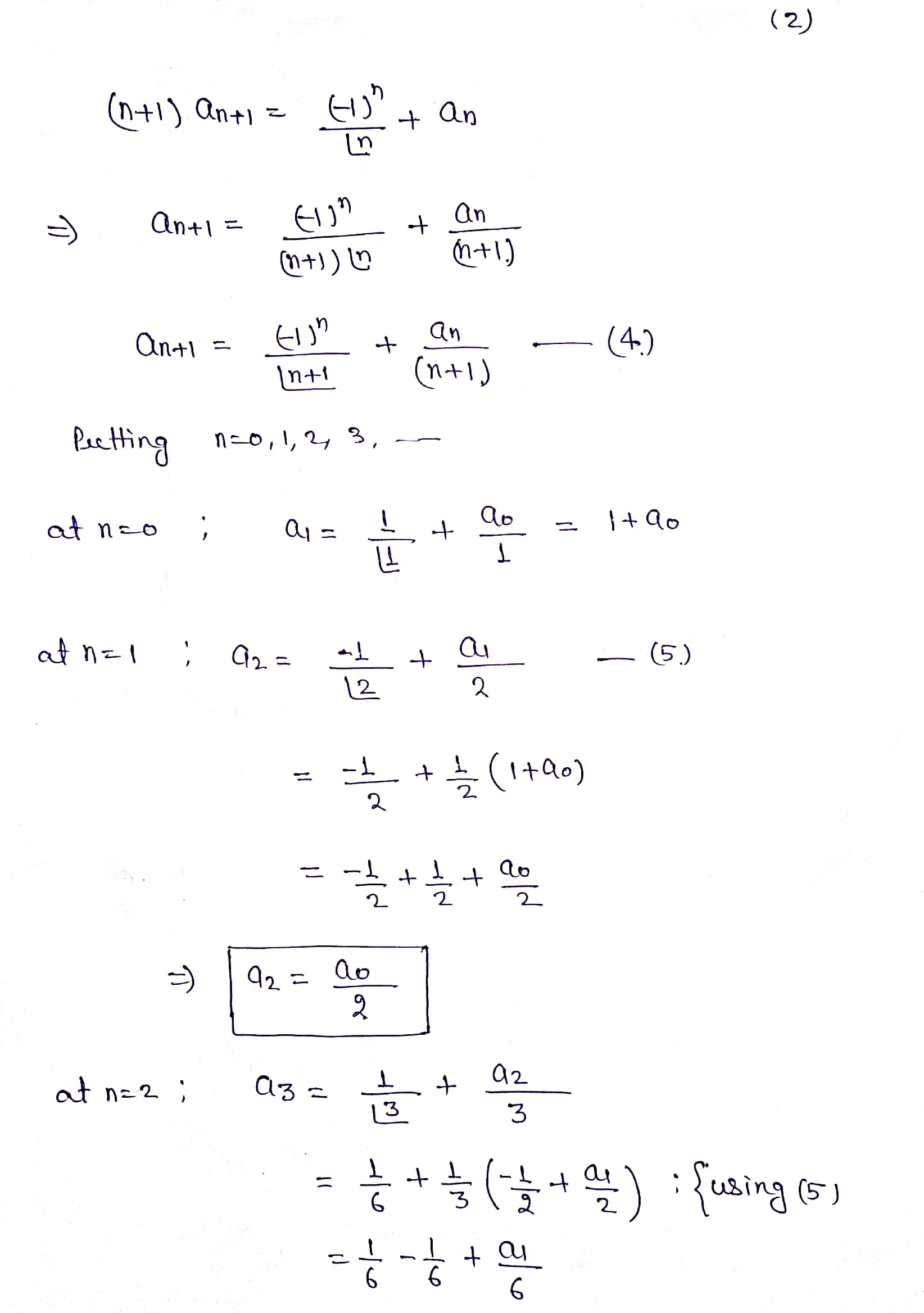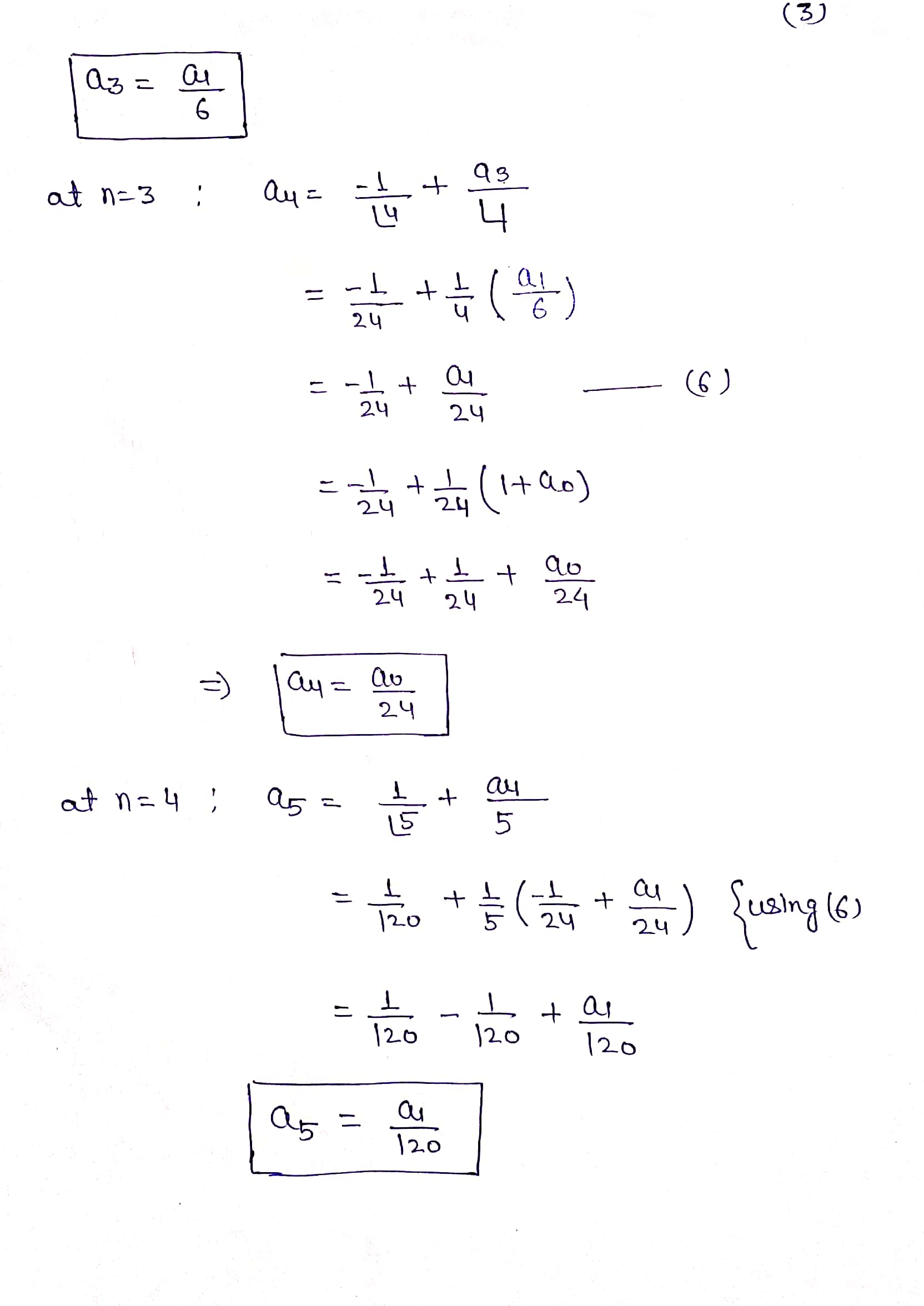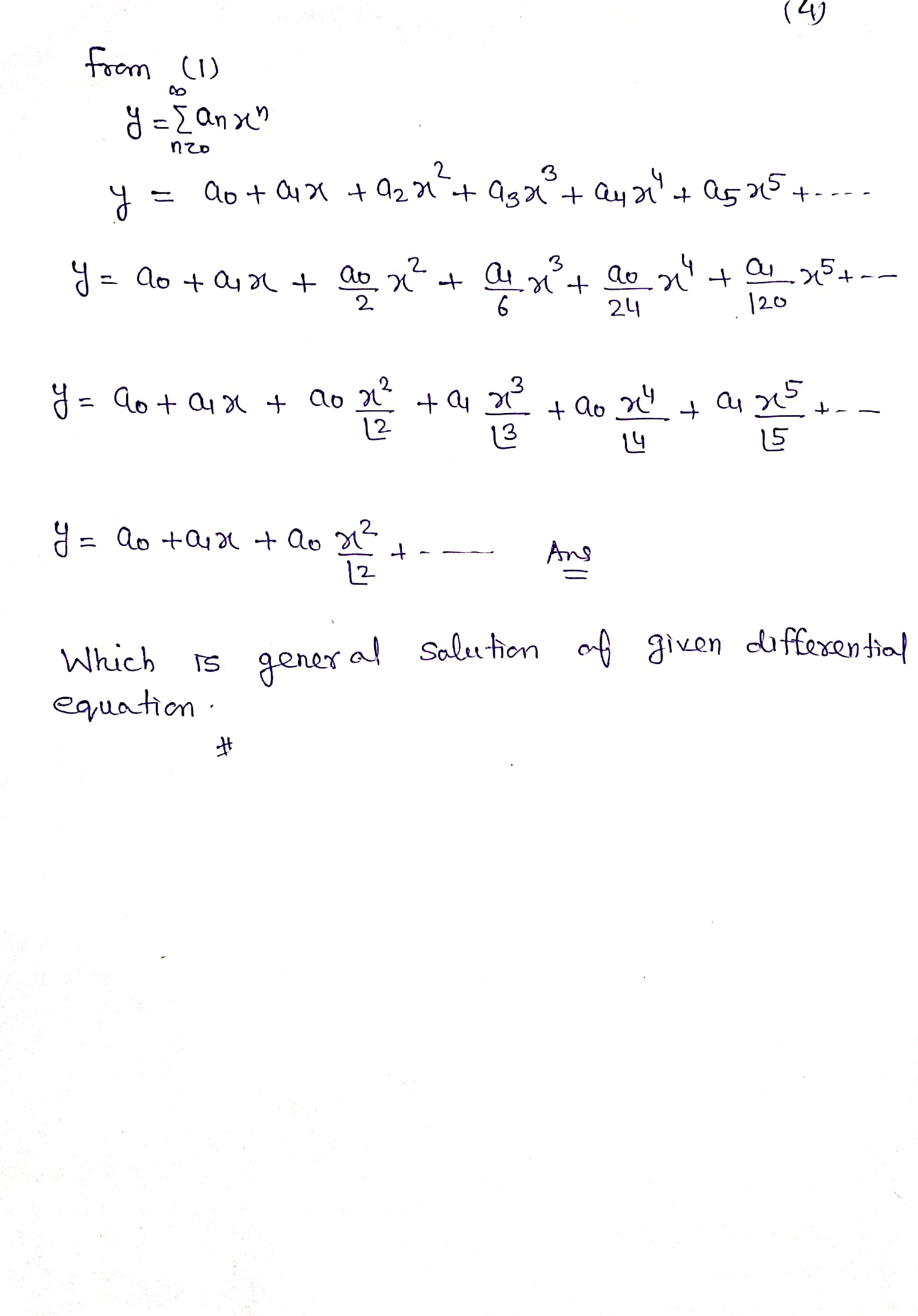##### Add Answer of: 4. Find the first three nonzero terms in a power series expansion about xo = 0...
Similar Homework Help Questions
• ### 1. Find the first three nonzero terms in a power series expansion about Xo = 0...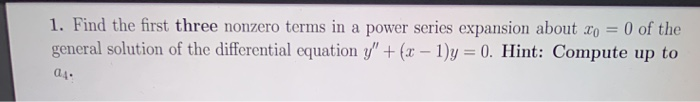1. Find the first three nonzero terms in a power series expansion about Xo = 0 of the general solution of the differential equation y" + (x - 1)y = 0. Hint: Compute up to 04.

• ### 1. Find the first three nonzero terms in a power series expansion about Xo = 0...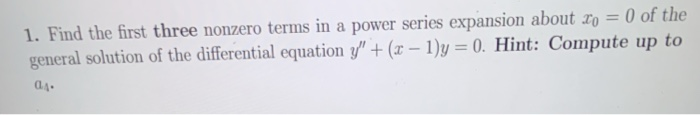1. Find the first three nonzero terms in a power series expansion about Xo = 0 of the general solution of the differential equation y" + (x - 1)y = 0. Hint: Compute up to 01.

• ### 3. Find the first three nonzero terms in a power series expansion about to 1 of...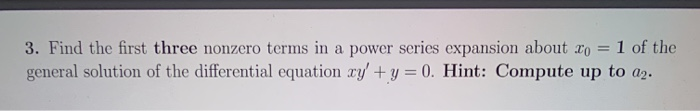3. Find the first three nonzero terms in a power series expansion about to 1 of the general solution of the differential equation xy + y = 0. Hint: Compute up to a2.

• ### Find the first four nonzero terms in a power series expansion about xo for a general...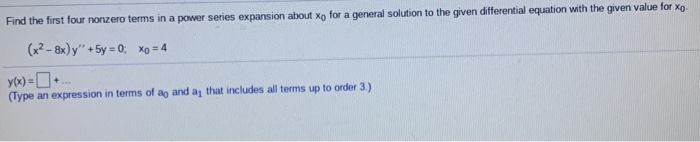Find the first four nonzero terms in a power series expansion about xo for a general solution to the given differential equation with the given value for Xo. (x2 - 8x y + 5y = 0, xo = 4 (Type an expression in terms of ag and a, that includes all terms up to order 3.)

• ### 8.4.9 Question Help Find the first four nonzero terms in a power series expansion about xo...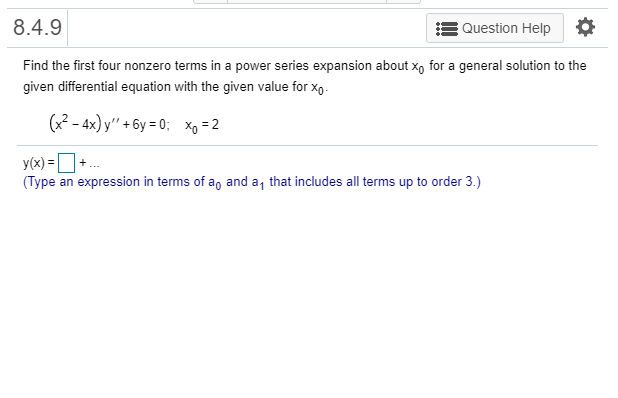8.4.9 Question Help Find the first four nonzero terms in a power series expansion about xo for a general solution to the given differential equation with the given value for Xo- (x2 - 4x) y" + 6y = 0; xp = 2 + y(x)= (Type an expression in terms of a, and a that includes all terms up to order 3.)

• ### 2. Find the first three nonzero terms in a power series expansion about to = 0...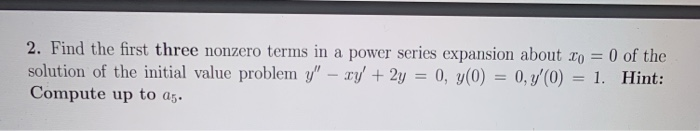2. Find the first three nonzero terms in a power series expansion about to = 0 of the solution of the initial value problem y" - xy + 2y = 0, y(0) = 0,7'0) = 1. Hint: Compute up to 25.

• ### X 8.4.11 Question Help Find the first four nonzero terms in a power series expansion about...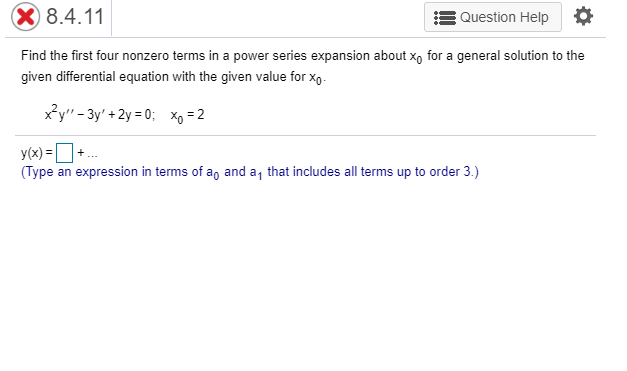X 8.4.11 Question Help Find the first four nonzero terms in a power series expansion about xo for a general solution to the given differential equation with the given value for Xo- x?y" - 3y' +2y = 0; Xo = 2 y(x)=+ (Type an expression in terms of a, and a that includes all terms up to order 3.)

• ### Find the first four nonzero terms in a power series expansion about xo - 2 for the solution to th...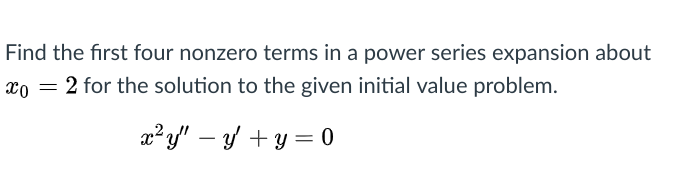Differential equations Find the first four nonzero terms in a power series expansion about xo - 2 for the solution to the given initial value problem Find the first four nonzero terms in a power series expansion about xo - 2 for the solution to the given initial value problem

• ### 8.3.14 Find the first four nonzero terms in a power series expansion about x = 0...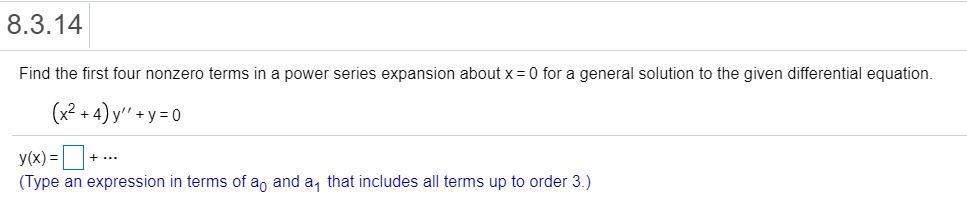8.3.14 Find the first four nonzero terms in a power series expansion about x = 0 for a general solution to the given differential equation. (x2 + 4) y'' +y=0 + ... y(x) = (Type an expression in terms of a, and a that includes all terms up to order 3.)

• ### 8.3.11 Find the first four nonzero terms in a power series expansion about x = 0...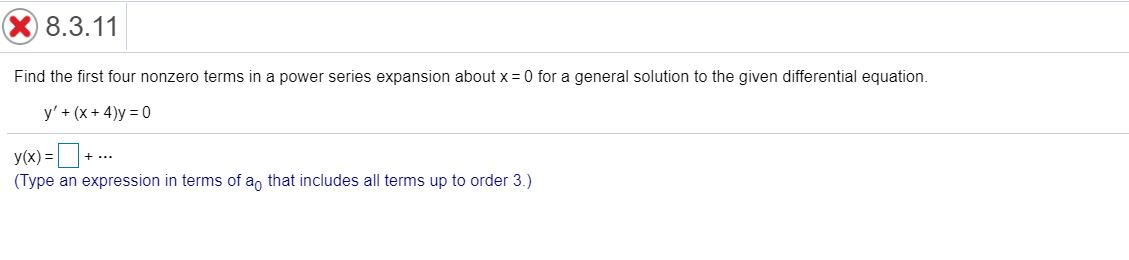8.3.11 Find the first four nonzero terms in a power series expansion about x = 0 for a general solution to the given differential equation. y' + (x + 4)y=0 +... y(x)=+. (Type an expression in terms of a, that includes all terms up to order 3.)

Free Homework App